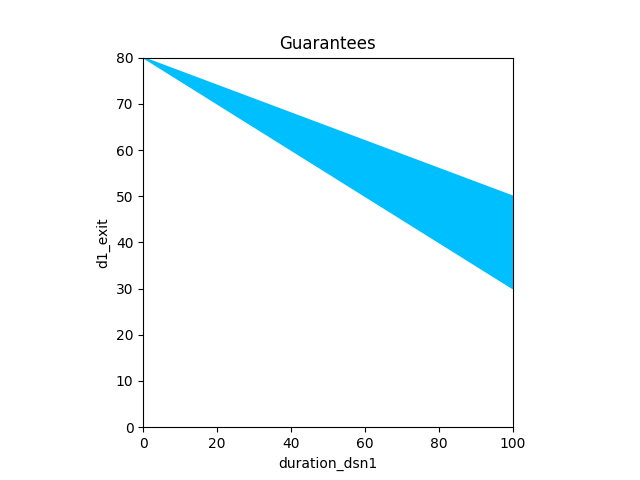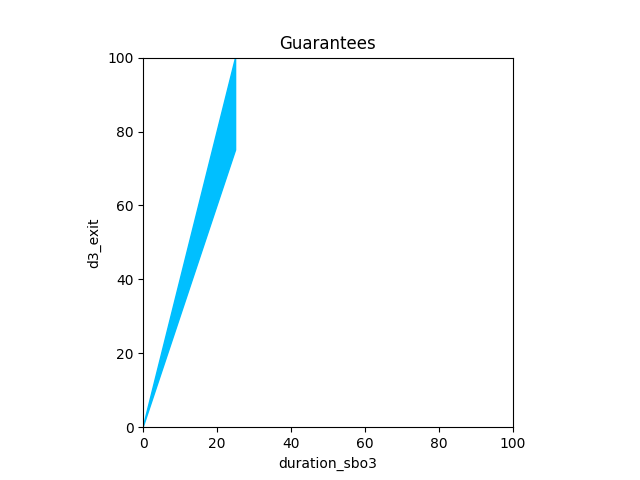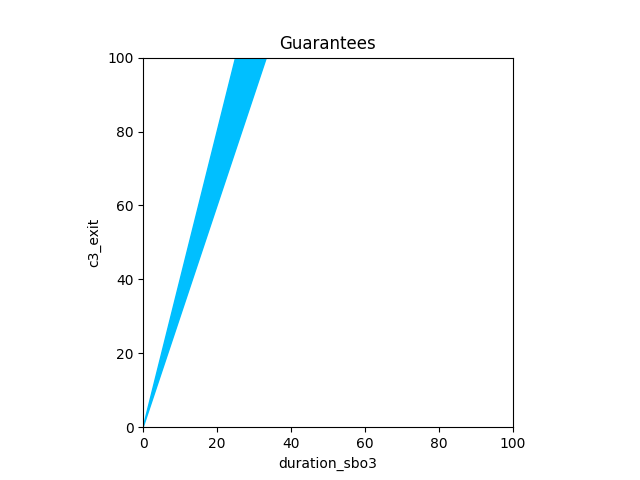# Science & Communication Viewpoint

%matplotlib widget

from pacti import write_contracts_to_file
from pacti.contracts import PolyhedralIoContract
from pacti.iocontract import Var
from contract_utils import *
from plot_utils import plot_guarantees_with_bounds_hover


## Science & Communication viewpoint modeling¶

Objective: charge the spacecraft battery

As summarized in the qualitative impacts table, this function has no science or communication effect.

charging1_science = nochange_contract(s=2, name="d").merge(nochange_contract(s=2, name="c"))
print(f"charging1_science:\n\n{charging1_science}")

charging1_science:

InVars: [d2_entry, c2_entry]
OutVars:[d2_exit, c2_exit]
A: [
-d2_entry <= -0
-c2_entry <= -0
]
G: [
-d2_entry + d2_exit = 0
-c2_entry + c2_exit = 0
]


Objective: downlink science data to Earth.

As summarized in the qualitative impacts table, this function affects this viewpoint with impacts that are linear with the duration of the task: - the onboard science data storage decreases proportionally to a downlink speed.

Note that this task has no impact on the cumulative science data.

# - s: start index of the timeline variables
def DSN_data(s: int, speed: Tuple[float, float]) -> PolyhedralIoContract:
spec = PolyhedralIoContract.from_strings(
input_vars = [
f"d{s}_entry",        # initial data volume
],
output_vars = [
f"d{s}_exit",          # final data volume
],
assumptions = [
# Task has a positive scheduled duration
f"duration_dsn{s} >= 0",

f"0 <= d{s}_entry <= 100",
],
guarantees = [
# duration*speed(min) <= d{entry} - d{exit} <= duration*speed(max)
f"{speed}*duration_dsn{s} <= d{s}_entry - d{s}_exit <= {speed}*duration_dsn{s}",

# downlink cannot continue if there is no data left.
f"d{s}_exit >= 0",
])
return spec

dsn1_science_storage = DSN_data(s=1, speed=(0.3, 0.5))
print(f"Contract dsn1_science_storage:\n\n{dsn1_science_storage}")
print('\n'.join(bounds(dsn1_science_storage)))

_ = plot_guarantees_with_bounds_hover(contract=dsn1_science_storage,
x_var=Var("duration_dsn1"),
y_var=Var("d1_exit"),
var_values={
Var("d1_entry"):80,
},
x_lims=(0,100),
y_lims=(0,80))

Contract dsn1_science_storage:

InVars: [d1_entry, duration_dsn1]
OutVars:[d1_exit]
A: [
-duration_dsn1 <= -0
-d1_entry <= -0
d1_entry <= 100
]
G: [
-d1_entry + d1_exit + 0.3 duration_dsn1 <= 0
d1_entry - d1_exit - 0.5 duration_dsn1 <= 0
-d1_exit <= 0
]
input d1_entry in [0.00,100.00]
input duration_dsn1 in [0.00,333.33]
output d1_exit in [0.00,100.00]

Figuredsn1_science_cumulative = nochange_contract(s=1, name="c")
print(f"dsn1_science_cumulative:\n\n{dsn1_science_cumulative}")

dsn1_science_cumulative:

InVars: [c1_entry]
OutVars:[c1_exit]
A: [
-c1_entry <= -0
]
G: [
-c1_entry + c1_exit = 0
]

dsn1_science = dsn1_science_storage.merge(dsn1_science_cumulative)
print(f"dsn1_science:\n\n{dsn1_science}")
print('\n'.join(bounds(dsn1_science)))

dsn1_science:

InVars: [d1_entry, duration_dsn1, c1_entry]
OutVars:[d1_exit, c1_exit]
A: [
-duration_dsn1 <= -0
-d1_entry <= -0
d1_entry <= 100
-c1_entry <= -0
]
G: [
-d1_entry + d1_exit + 0.3 duration_dsn1 <= 0
d1_entry - d1_exit - 0.5 duration_dsn1 <= 0
-d1_exit <= 0
-c1_entry + c1_exit = 0
]
input c1_entry in [0.00,None]
input d1_entry in [0.00,100.00]
input duration_dsn1 in [0.00,333.33]
output c1_exit in [0.00,None]
output d1_exit in [0.00,100.00]


### SBO Task (Small body observations)¶

Objective: Acquire small body observations (science data & navigation)

As summarized in the qualitative impacts table, this function affects this viewpoint with impacts that are linear with the duration of the task: - the science data storage grows proportionally to a generation rate. - the cumulative science data grows proportionally at the same rate.

# Parameters:
# - s: start index of the timeline variables
# - generation: (min, max) rate of small body observations during the task instance
def SBO_science_storage(s: int, generation: Tuple[float, float]) -> PolyhedralIoContract:
spec = PolyhedralIoContract.from_strings(
input_vars = [
f"d{s}_entry",        # initial data storage volume
f"duration_sbo{s}",   # knob variable for SBO duration
],
output_vars = [
f"d{s}_exit",         # final data storage volume
],
assumptions = [
# Task has a positive scheduled duration
f"duration_sbo{s} >= 0",

# There is enough data storage available
f"d{s}_entry + {generation}*duration_sbo{s} <= 100",

f"d{s}_entry >= 0",
],
guarantees = [
# duration*generation(min) <= d{exit} - d{entry} <= duration*generation(max)
f"{generation}*duration_sbo{s} <= d{s}_exit - d{s}_entry <= {generation}*duration_sbo{s}",

# Data volume cannot exceed the available storage capacity
f"d{s}_exit <= 100",
])
return spec

sbo1_science_storage = SBO_science_storage(s=3, generation=(3.0, 4.0))
print(f"Contract sbo1_science_storage:\n\n{sbo1_science_storage}")
print('\n'.join(bounds(sbo1_science_storage)))

_ = plot_guarantees_with_bounds_hover(contract=sbo1_science_storage,
x_var=Var("duration_sbo3"),
y_var=Var("d3_exit"),
var_values={
Var("d3_entry"):0,
},
x_lims=(0,100),
y_lims=(0,100))

Contract sbo1_science_storage:

InVars: [d3_entry, duration_sbo3]
OutVars:[d3_exit]
A: [
-duration_sbo3 <= -0
d3_entry + 4 duration_sbo3 <= 100
-d3_entry <= -0
]
G: [
d3_entry - d3_exit + 3 duration_sbo3 <= 0
-d3_entry + d3_exit - 4 duration_sbo3 <= 0
]
input d3_entry in [0.00,100.00]
input duration_sbo3 in [0.00,25.00]
output d3_exit in [0.00,100.00]

Figuredef SBO_science_comulative(s: int, generation: Tuple[float, float]) -> PolyhedralIoContract:
spec = PolyhedralIoContract.from_strings(
input_vars = [
f"c{s}_entry",        # initial cumulative data volume
f"duration_sbo{s}",   # knob variable for SBO duration
],
output_vars = [
f"c{s}_exit",         # final cumulative data volume
],
assumptions = [
# Task has a positive scheduled duration
f"duration_sbo{s} >= 0",

# cumulative data lower bound
f"c{s}_entry >= 0",
],
guarantees = [
# duration*generation(min) <= c{exit} - c{entry} <= duration*generation(max)
f"{generation}*duration_sbo{s} <= c{s}_exit - c{s}_entry <= {generation}*duration_sbo{s}",
])
return spec

sbo1_science_cumulative = SBO_science_comulative(s=3, generation=(3.0, 4.0))
print(f"Contract sbo1_science_cumulative:\n\n{sbo1_science_cumulative}")
print('\n'.join(bounds(sbo1_science_cumulative)))

_ = plot_guarantees_with_bounds_hover(contract=sbo1_science_cumulative,
x_var=Var("duration_sbo3"),
y_var=Var("c3_exit"),
var_values={
Var("c3_entry"):0,
},
x_lims=(0,100),
y_lims=(0,100))

Contract sbo1_science_cumulative:

InVars: [c3_entry, duration_sbo3]
OutVars:[c3_exit]
A: [
-duration_sbo3 <= -0
-c3_entry <= -0
]
G: [
c3_entry - c3_exit + 3 duration_sbo3 <= 0
-c3_entry + c3_exit - 4 duration_sbo3 <= 0
]
input c3_entry in [0.00,None]
input duration_sbo3 in [0.00,None]
output c3_exit in [0.00,None]

Figuresbo1_science = sbo1_science_storage.merge(sbo1_science_cumulative)
print(f"Contract sbo1_science:\n\n{sbo1_science}")
print('\n'.join(bounds(sbo1_science)))

Contract sbo1_science:

InVars: [d3_entry, duration_sbo3, c3_entry]
OutVars:[d3_exit, c3_exit]
A: [
-duration_sbo3 <= -0
d3_entry + 4 duration_sbo3 <= 100
-d3_entry <= -0
-c3_entry <= -0
]
G: [
d3_entry - d3_exit + 3 duration_sbo3 <= 0
-d3_entry + d3_exit - 4 duration_sbo3 <= 0
c3_entry - c3_exit + 3 duration_sbo3 <= 0
-c3_entry + c3_exit - 4 duration_sbo3 <= 0
]
input c3_entry in [0.00,None]
input d3_entry in [0.00,100.00]
input duration_sbo3 in [0.00,25.00]
output c3_exit in [0.00,None]
output d3_exit in [0.00,100.00]


### TCM Task (Perform a Trajectory Correction Maneuver)¶

Objective: Perform a delta-V maneuver to bring the spacecraft trajectory closer to that of the small body.

As described in the qualitative impacts table, this function has no impact on science.

Since TCM Heating has no impact on navigation, we use the no-change contract utility to specify this property for the two science viewpoint state variables: d and c.

tcm1_science_heating = nochange_contract(s=4, name="d").merge(nochange_contract(s=4, name="c"))
print(f"tcm1_science_heating:\n{tcm1_science_heating}")

tcm1_science_heating:
InVars: [d4_entry, c4_entry]
OutVars:[d4_exit, c4_exit]
A: [
-d4_entry <= -0
-c4_entry <= -0
]
G: [
-d4_entry + d4_exit = 0
-c4_entry + c4_exit = 0
]

tcm1_science_deltav = nochange_contract(s=5, name="d").merge(nochange_contract(s=5, name="c"))
print(f"tcm1_science_deltav:\n{tcm1_science_deltav}")

tcm1_science_deltav:
InVars: [d5_entry, c5_entry]
OutVars:[d5_exit, c5_exit]
A: [
-d5_entry <= -0
-c5_entry <= -0
]
G: [
-d5_entry + d5_exit = 0
-c5_entry + c5_exit = 0
]

tcm1_science = scenario_sequence(c1=tcm1_science_heating, c2=tcm1_science_deltav, variables=["d", "c"], c1index=4)
print(f"tcm1_science:\n{tcm1_science}")
print('\n'.join(bounds(tcm1_science)))

tcm1_science:
InVars: [d4_entry, c4_entry]
OutVars:[d5_exit, c5_exit, output_d4, output_c4]
A: [
-d4_entry <= 0
-c4_entry <= 0
]
G: [
-d4_entry + output_d4 = 0
-c4_entry + output_c4 = 0
d5_exit - output_d4 = 0
c5_exit - output_c4 = 0
]
input c4_entry in [0.00,None]
input d4_entry in [0.00,None]
output c5_exit in [0.00,None]
output d5_exit in [0.00,None]
output output_c4 in [0.00,None]
output output_d4 in [0.00,None]


### science & Communication Schedule Analysis¶

Let's consider a simple 4-step schedule of the following sequence of task instances, which we compose: - DSN - CHARGING - SBO - TCM

print(dsn1_science)

InVars: [d1_entry, duration_dsn1, c1_entry]
OutVars:[d1_exit, c1_exit]
A: [
-duration_dsn1 <= -0
-d1_entry <= -0
d1_entry <= 100
-c1_entry <= -0
]
G: [
-d1_entry + d1_exit + 0.3 duration_dsn1 <= 0
d1_entry - d1_exit - 0.5 duration_dsn1 <= 0
-d1_exit <= 0
-c1_entry + c1_exit = 0
]

steps12=scenario_sequence(c1=dsn1_science, c2=charging1_science, variables=["d", "c"], c1index=1)
print(f"---- Steps 1,2\n{steps12}")
print('\n'.join(bounds(steps12)))

---- Steps 1,2
InVars: [d1_entry, duration_dsn1, c1_entry]
OutVars:[d2_exit, c2_exit, output_d1, output_c1]
A: [
-duration_dsn1 <= 0
-d1_entry <= 0
d1_entry <= 100
-c1_entry <= 0
]
G: [
-d1_entry + 0.3 duration_dsn1 + output_d1 <= 0
d1_entry - 0.5 duration_dsn1 - output_d1 <= 0
-output_d1 <= 0
-c1_entry + output_c1 = 0
d2_exit - output_d1 = 0
c2_exit - output_c1 = 0
]
input c1_entry in [0.00,None]
input d1_entry in [0.00,100.00]
input duration_dsn1 in [0.00,333.33]
output c2_exit in [0.00,None]
output d2_exit in [0.00,100.00]
output output_c1 in [0.00,None]
output output_d1 in [0.00,100.00]

steps123=scenario_sequence(c1=steps12, c2=sbo1_science, variables=["d", "c"], c1index=2)
print(f"---- Steps 1,2,3\n{steps123}")
print('\n'.join(bounds(steps123)))

---- Steps 1,2,3
InVars: [d1_entry, duration_dsn1, c1_entry, duration_sbo3]
OutVars:[output_d1, output_c1, d3_exit, c3_exit, output_d2, output_c2]
A: [
-duration_sbo3 <= 0
d1_entry - 0.3 duration_dsn1 + 4 duration_sbo3 <= 100
-duration_dsn1 <= 0
-d1_entry <= 0
d1_entry <= 100
-c1_entry <= 0
]
G: [
-d1_entry + 0.3 duration_dsn1 + output_d1 <= 0
d1_entry - 0.5 duration_dsn1 - output_d1 <= 0
-output_d1 <= 0
-c1_entry + output_c1 = 0
-output_d1 + output_d2 = 0
-output_c1 + output_c2 = 0
-d3_exit + 3 duration_sbo3 + output_d2 <= 0
d3_exit - 4 duration_sbo3 - output_d2 <= 0
-c3_exit + 3 duration_sbo3 + output_c2 <= 0
c3_exit - 4 duration_sbo3 - output_c2 <= 0
]
input c1_entry in [0.00,None]
input d1_entry in [0.00,100.00]
input duration_dsn1 in [0.00,333.33]
input duration_sbo3 in [0.00,25.00]
output c3_exit in [0.00,None]
output d3_exit in [0.00,100.00]
output output_c1 in [0.00,None]
output output_c2 in [0.00,None]
output output_d1 in [0.00,100.00]
output output_d2 in [0.00,100.00]

steps1234=scenario_sequence(c1=steps123, c2=tcm1_science, variables=["d", "c"], c1index=3)
print(f"---- Steps 1,2,3,4\n{steps1234}")

---- Steps 1,2,3,4
InVars: [d1_entry, duration_dsn1, c1_entry, duration_sbo3]
OutVars:[output_d1, output_c1, output_d2, output_c2, d5_exit, c5_exit, output_d4, output_c4, output_d3, output_c3]
A: [
-duration_sbo3 <= 0
d1_entry - 0.3 duration_dsn1 + 4 duration_sbo3 <= 100
-duration_dsn1 <= 0
-d1_entry <= 0
d1_entry <= 100
-c1_entry <= 0
]
G: [
-d1_entry + 0.3 duration_dsn1 + output_d1 <= 0
d1_entry - 0.5 duration_dsn1 - output_d1 <= 0
-output_d1 <= 0
-c1_entry + output_c1 = 0
-output_d1 + output_d2 = 0
-output_c1 + output_c2 = 0
3 duration_sbo3 + output_d2 - output_d3 <= 0
-4 duration_sbo3 - output_d2 + output_d3 <= 0
3 duration_sbo3 + output_c2 - output_c3 <= 0
-4 duration_sbo3 - output_c2 + output_c3 <= 0
-output_d3 + output_d4 = 0
-output_c3 + output_c4 = 0
d5_exit - output_d4 = 0
c5_exit - output_c4 = 0
]

scenario_science=steps1234.rename_variables([
("d4_exit", "output_d4"),
("c4_exit", "output_c4"),
("d5_exit", "output_d5"),
("c5_exit", "output_c5")])
print(f"scenario_science={scenario_science}")
print('\n'.join(bounds(scenario_science)))

scenario_science=InVars: [d1_entry, duration_dsn1, c1_entry, duration_sbo3]
OutVars:[output_d1, output_c1, output_d2, output_c2, output_d4, output_c4, output_d3, output_c3, output_d5, output_c5]
A: [
-duration_sbo3 <= 0
d1_entry - 0.3 duration_dsn1 + 4 duration_sbo3 <= 100
-duration_dsn1 <= 0
-d1_entry <= 0
d1_entry <= 100
-c1_entry <= 0
]
G: [
-d1_entry + 0.3 duration_dsn1 + output_d1 <= 0
d1_entry - 0.5 duration_dsn1 - output_d1 <= 0
-output_d1 <= 0
-c1_entry + output_c1 = 0
-output_d1 + output_d2 = 0
-output_c1 + output_c2 = 0
3 duration_sbo3 + output_d2 - output_d3 <= 0
-4 duration_sbo3 - output_d2 + output_d3 <= 0
3 duration_sbo3 + output_c2 - output_c3 <= 0
-4 duration_sbo3 - output_c2 + output_c3 <= 0
-output_d3 + output_d4 = 0
-output_c3 + output_c4 = 0
-output_d4 + output_d5 = 0
-output_c4 + output_c5 = 0
]
input c1_entry in [0.00,None]
input d1_entry in [0.00,100.00]
input duration_dsn1 in [0.00,333.33]
input duration_sbo3 in [0.00,25.00]
output output_c1 in [0.00,None]
output output_c2 in [0.00,None]
output output_c3 in [0.00,None]
output output_c4 in [0.00,None]
output output_c5 in [0.00,None]
output output_d1 in [0.00,100.00]
output output_d2 in [0.00,100.00]
output output_d3 in [0.00,100.00]
output output_d4 in [0.00,100.00]
output output_d5 in [0.00,100.00]

back34=scenario_sequence(c1=sbo1_science, c2=tcm1_science, variables=["d", "c"], c1index=3)
print(f"---- R2L Steps 3,4\n{back34}")
print('\n'.join(bounds(back34)))

---- R2L Steps 3,4
InVars: [d3_entry, duration_sbo3, c3_entry]
OutVars:[d5_exit, c5_exit, output_d4, output_c4, output_d3, output_c3]
A: [
-duration_sbo3 <= 0
d3_entry + 4 duration_sbo3 <= 100
-d3_entry <= 0
-c3_entry <= 0
]
G: [
d3_entry + 3 duration_sbo3 - output_d3 <= 0
-d3_entry - 4 duration_sbo3 + output_d3 <= 0
c3_entry + 3 duration_sbo3 - output_c3 <= 0
-c3_entry - 4 duration_sbo3 + output_c3 <= 0
-output_d3 + output_d4 = 0
-output_c3 + output_c4 = 0
d5_exit - output_d4 = 0
c5_exit - output_c4 = 0
]
input c3_entry in [0.00,None]
input d3_entry in [0.00,100.00]
input duration_sbo3 in [0.00,25.00]
output c5_exit in [0.00,None]
output d5_exit in [0.00,100.00]
output output_c3 in [0.00,None]
output output_c4 in [0.00,None]
output output_d3 in [0.00,100.00]
output output_d4 in [0.00,100.00]

back234=scenario_sequence(c1=charging1_science, c2=back34, variables=["d", "c"], c1index=2)
print(f"---- R2L Steps 2,3,4\n{back234}")
print('\n'.join(bounds(back234)))

---- R2L Steps 2,3,4
InVars: [d2_entry, c2_entry, duration_sbo3]
OutVars:[d5_exit, c5_exit, output_d4, output_c4, output_d3, output_c3, output_d2, output_c2]
A: [
-duration_sbo3 <= 0
d2_entry + 4 duration_sbo3 <= 100
-d2_entry <= 0
-c2_entry <= 0
]
G: [
-d2_entry + output_d2 = 0
-c2_entry + output_c2 = 0
3 duration_sbo3 + output_d2 - output_d3 <= 0
-4 duration_sbo3 - output_d2 + output_d3 <= 0
3 duration_sbo3 + output_c2 - output_c3 <= 0
-4 duration_sbo3 - output_c2 + output_c3 <= 0
-output_d3 + output_d4 = 0
-output_c3 + output_c4 = 0
d5_exit - output_d4 = 0
c5_exit - output_c4 = 0
]
input c2_entry in [0.00,None]
input d2_entry in [0.00,100.00]
input duration_sbo3 in [0.00,25.00]
output c5_exit in [0.00,None]
output d5_exit in [0.00,100.00]
output output_c2 in [0.00,None]
output output_c3 in [0.00,None]
output output_c4 in [0.00,None]
output output_d2 in [0.00,100.00]
output output_d3 in [0.00,100.00]
output output_d4 in [0.00,100.00]

back1234=scenario_sequence(c1=dsn1_science, c2=back234, variables=["d", "c"], c1index=1).rename_variables([
("d4_exit", "output_d4"),
("c4_exit", "output_c4"),
("d5_exit", "output_d5"),
("c5_exit", "output_c5")])
print(f"---- R2L Steps 1,2,3,4\n{back1234}")
print('\n'.join(bounds(back1234)))

---- R2L Steps 1,2,3,4
InVars: [d1_entry, duration_dsn1, c1_entry, duration_sbo3]
OutVars:[output_d4, output_c4, output_d3, output_c3, output_d2, output_c2, output_d1, output_c1, output_d5, output_c5]
A: [
-duration_sbo3 <= 0
d1_entry - 0.3 duration_dsn1 + 4 duration_sbo3 <= 100
-duration_dsn1 <= 0
-d1_entry <= 0
d1_entry <= 100
-c1_entry <= 0
]
G: [
-d1_entry + 0.3 duration_dsn1 + output_d1 <= 0
d1_entry - 0.5 duration_dsn1 - output_d1 <= 0
-output_d1 <= 0
-c1_entry + output_c1 = 0
-output_d1 + output_d2 = 0
-output_c1 + output_c2 = 0
3 duration_sbo3 + output_d2 - output_d3 <= 0
-4 duration_sbo3 - output_d2 + output_d3 <= 0
3 duration_sbo3 + output_c2 - output_c3 <= 0
-4 duration_sbo3 - output_c2 + output_c3 <= 0
-output_d3 + output_d4 = 0
-output_c3 + output_c4 = 0
-output_d4 + output_d5 = 0
-output_c4 + output_c5 = 0
]
input c1_entry in [0.00,None]
input d1_entry in [0.00,100.00]
input duration_dsn1 in [0.00,333.33]
input duration_sbo3 in [0.00,25.00]
output output_c1 in [0.00,None]
output output_c2 in [0.00,None]
output output_c3 in [0.00,None]
output output_c4 in [0.00,None]
output output_c5 in [0.00,None]
output output_d1 in [0.00,100.00]
output output_d2 in [0.00,100.00]
output output_d3 in [0.00,100.00]
output output_d4 in [0.00,100.00]
output output_d5 in [0.00,100.00]

write_contracts_to_file(
contracts=[scenario_science, back1234],
names=["scenario_science_l2r", "scenario_science_r2l"],
file_name="json/scenario_science.json")